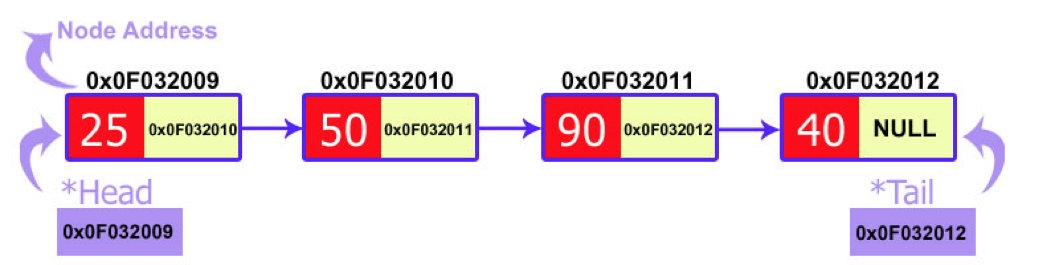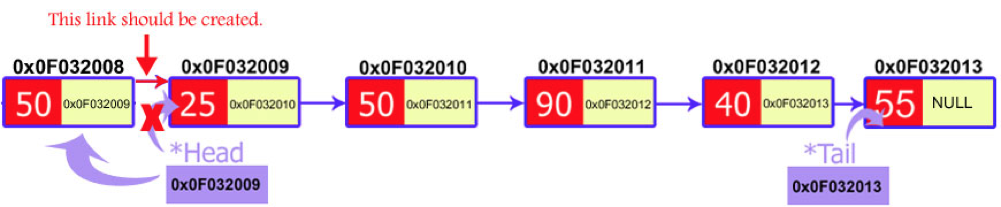### Introduction

Linked lists are the best and simplest example of a dynamic data structure that uses pointers for its implementation. However, understanding pointers is crucial to understanding how linked lists work, so if you've skipped the pointers tutorial, you should go back and redo it. You must also be familiar with dynamic memory allocation and structures.

Essentially, linked lists function as an array that can grow and shrink as needed, from any point in the array.

1. Items can be added or removed from the middle of the list
2. There is no need to define an initial size

1. There is no "random" access - it is impossible to reach the nth item in the array without first iterating over all items up until that item. This means we have to start from the beginning of the list and count how many times we advance in the list until we get to the desired item.
2. Dynamic memory allocation and pointers are required, which complicates the code and increases the risk of memory leaks and segment faults.
3. Linked lists have a much larger overhead over arrays, since linked list items are dynamically allocated (which is less efficient in memory usage) and each item in the list also must store an additional pointer.

### What is a linked list?

A linked list is a set of dynamically allocated nodes, arranged in such a way that each node contains one value and one pointer. The pointer always points to the next member of the list. If the pointer is `nullptr`, then it is the last node in the list.Let's define a linked list node:

``````struct Node {
int value;
struct Node * next;
};
``````

A linked list is held using a pointer which points to the first item of the linked list called "head" and a pointer which points to the last item of the linked list called "tail". If that pointer (the "tail") is also `nullptr`, then the list is considered to be empty.

``````class LinkedList
{
public:
{
tail = nullptr;
}

private:
Node *tail;
};
``````

Now we can use the nodes. Let's create a method `createNode` to create the first item of the linked list. The process of creating node is as follows. We need a pointer of a `Node` type (which we defined) and we will insert the value in its data field. The next field of `Node` would be declared as `nullptr` as it would be the last node of linked list.

There is a special case, which we need to check for namely when the linked list is empty. As we know, `head` points to the first node? It means if the `head` is equal to `nullptr` then we can conclude that the linked list is empty.

If there is just one node (which we are going to create) in a linked lists, then both `head` and `tail` will point to this element.

If a linked list is already created, the new node should be inserted at the end of the linked list. We know that `tail` points to the last node. Therefore, the newly created node will be next to the node `tail` is pointing to.

The creation of a new node at the end of linked list has two steps:

1. Linking the newly created node to `tail`. Means passing the address of a new node to the next pointer of `tail`.

2. The `tail` pointer should always point to the last node. So we will make our `tail` pointer equal to a new node.``````class LinkedList
{
public:
{
tail = nullptr;
}

void createNode(int value)
{
node *temp = new Node;
temp->data = value;
temp->next = nullptr;

{
tail = temp;
}
else
{
tail->next = temp;
tail = temp;
}
}

private:
Node *tail;
};
``````

### Iterating over a list

Let's build a function that prints out all the items of a list (`printList`). To do this, we need to use a `current` pointer that will keep track of the node we are currently printing. After printing the value of the node, we set the `current` pointer to the next node, and print again, until we've reached the end of the list (the next node is `nullptr`).

``````class LinkedList
{
public:
{
tail = nullptr;
}

void createNode(int value)
{
node *temp = new Node;
temp->data = value;
temp->next = nullptr;

{
tail = temp;
}
else
{
tail->next = temp;
tail = temp;
}
}

void printList() {

while (current != nullptr) {
std::cout << current->value << std::endl;
current = current->next;
}
}

private:
Node *tail;
};
``````

### Insertion

Inserting a new node in the linked list is called insertion.

A new node is created and inserted in the linked list.

There are three cases considered while inserting a node:

1. Insertion at the start
2. Insertion at the end
3. Insertion at a particular position

#### Insertion an item at the start of the list (pushing to the list)

To add to the beginning of the list, we will need to do the following three steps:

1. Create a new item and set its value
2. Link the new item to point to the `head` of the list
3. Set the `head` of the list to be our new itemThis will effectively create a new `head` to the list with a new value, and keep the rest of the list linked to it.

Since we use a method to do this operation, we want to be able to modify the `head` variable. To do this, we must pass a pointer to the pointer variable (a double pointer) so we will be able to modify the pointer itself.

``````void pushNode(int value) {
Node * new_node;
new_node = new Node;

new_node->value = value;

}
``````

#### Insertion at the End

The insertion of a node at the end of a linked list is the same as we have done in node creation function. If you noticed then, we inserted the newly created node at the end of the linked list. So this process is the same.

#### Insertion at Particular Position

The insertion of a new node at a particular position is slightly more difficult. In this case, we don’t disturb the `head` and `tail` nodes. Rather, a new node is inserted between two consecutive nodes. So, these two nodes should be accessible by our code. We call one node as `current` and the other as `previous`, and the new node is placed between them.

The new node can be inserted between the `previous` and `current` node by just performing two steps:

1. Pass the address of the new node in the next field of the previous node.
2. Pass the address of the current node in the next field of the new node.

We will access these nodes by asking the user at what position he wants to insert the new node. Then, we will start a loop to reach those specific nodes. We initialized our current node by the head and move through the linked list. At the end, we would find two consecutive nodes.

C++ code for insertion of node would be as follows:

``````void insertNodeAtPosition(int pos, int value)
{
Node *pre = new Node;
Node *current = new Node;
Node *temp = new Node;

for(int i = 1; i < pos; i++)
{
pre = current;
current = current->next;
}
temp->data = value;
pre->next = temp;
temp->next = current;
}
``````

### Deletion:

So, you have become familiar with linked list creation. Now, it’s time to do some manipulation on the linked list created. Linked lists provide us the great feature of deleting a node. The process of deletion is also easy to implement. The basic structure is to declare a temporary pointer which points the node to be deleted. Then a little bit of working on links of nodes. There are also three cases in which a node can be deleted:

1. Deletion at the start
2. Deletion at the end
3. Deletion at a particular position

### Deletion of the first item (popping from the linked list)

To pop a variable, we will need to perform three steps:

1. Take the next item that the `head` points to and save it
3. Set the head to be the next item that we've stored on the side

Here is the code:

``````int pop() {
int retval = 0;
Node * next_node = nullptr;

int retval;
}
``````

### Deletion of the last item of the list

Removing the last item from a list is very similar to adding it to the end of the list, but with one big exception - since we have to change one item before the last item, we actually have to look two items ahead and see if the next item is the last one in the list:

``````int removeLast() {
int retval = 0;
/* if there is only one item in the list, remove it */
return retval;
}

/* get to the second to last node in the list */
while (current->next->next != nullptr) {
current = current->next;
}

/* now current points to the second to last item of the list, so let's remove current->next */
retval = current->next->value;
delete current->next;
current->next = nullptr;
return retval;
}
``````

### Removing a specific item

To remove a specific item from the list, either by its index from the beginning of the list or by its value, we will need to go over all the items, continuously looking ahead to find out if we've reached the node before the item we wish to remove. This is because we need to change the location to where the previous node points to as well.

Here is the algorithm:

1. Iterate to the node before the node we wish to delete
2. Save the node we wish to delete in a temporary pointer
3. Set the previous node's next pointer to point to the node after the node we wish to delete
4. Delete the node using the temporary pointer

There are a few edge cases we need to take care of, so make sure you understand the code.

``````int removeByIndex(int n) {
int i = 0;
int retval = -1;
Node * temp_node = nullptr;

if (n == 0) {
}

for (i = 0; i < n-1; i++) {
if (current->next == nullptr) {
return -1;
}
current = current->next;
}

temp_node = current->next;
retval = temp_node->value;
current->next = temp_node->next;
delete temp_node;

return retval;

}
``````

## Exercise

You must implement the function `removeByValue` which removes the first item in the list which has the value `value`.